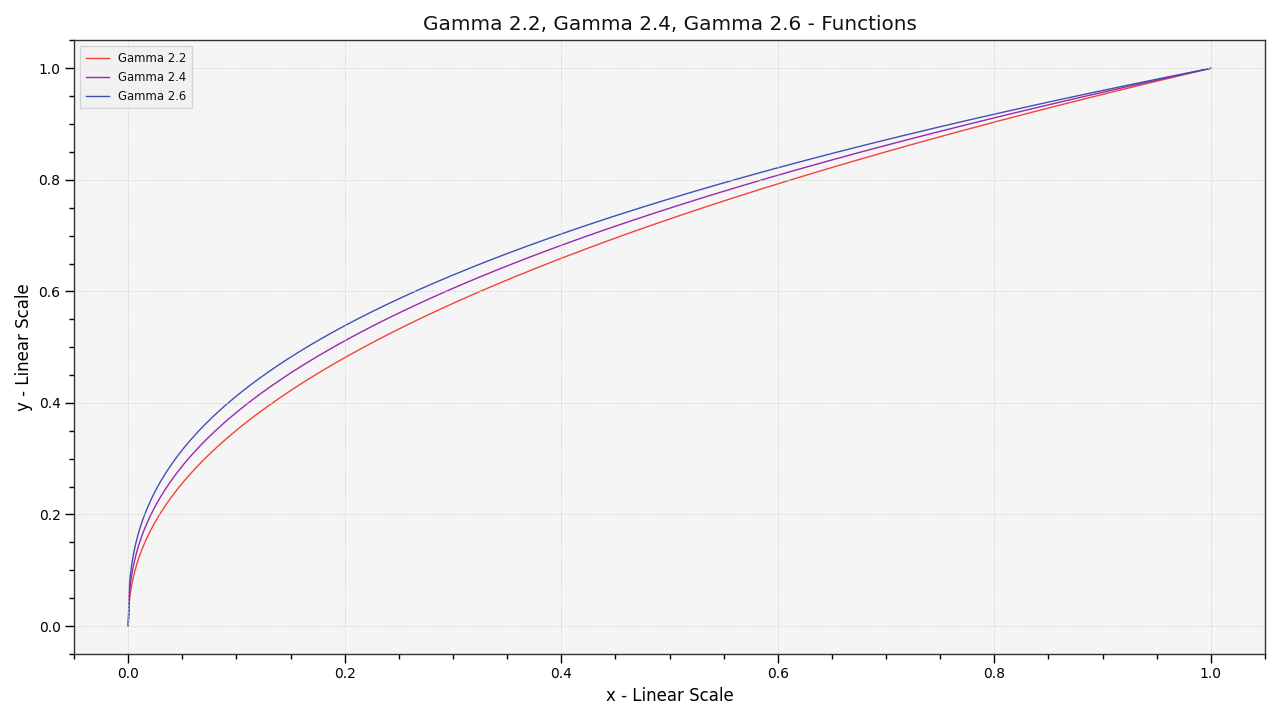# colour.plotting.plot_multi_functions#

colour.plotting.plot_multi_functions(functions: , samples: Optional[ArrayLike] = None, log_x: = None, log_y: = None, plot_kwargs: Optional[Union[dict, List[dict]]] = None, **kwargs: Any) [source]#

Plot given functions.

Parameters:
Returns:

Current figure and axes.

Return type:

`tuple`

Examples

```>>> functions = {
...     "Gamma 2.2": lambda x: x ** (1 / 2.2),
...     "Gamma 2.4": lambda x: x ** (1 / 2.4),
...     "Gamma 2.6": lambda x: x ** (1 / 2.6),
... }
>>> plot_multi_functions(functions)
...
(<Figure size ... with 1 Axes>, <...AxesSubplot...>)
```